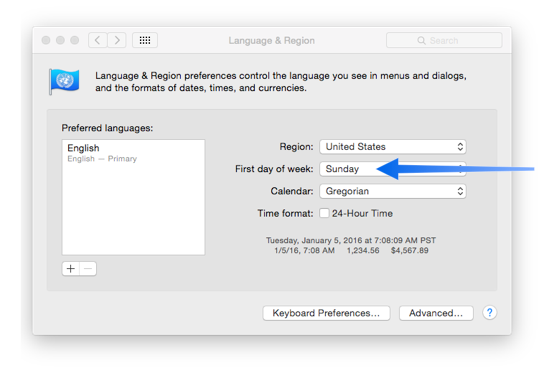week1st(
DATE
)

The week1st( function computes the first day of a week (Sunday).

Parameters

This function has one parameter:

date – is a number representing the date.

Description

This function calculates the first day of the week. For example, in the United States if the date passed to the function is July 12, 1995 (a Wednesday), it will return the date July 9, 1995 (a Sunday). The date is returned as a number.

``````datepattern(week1st(date("7/12/95")),"Month ddnth, YYYY") ☞ July 9th, 1995
``````

As stated above the first day of the week is usually Sunday in the United States, but it can be different in other parts of the world, and it can be changed in the System Preferences Language & Region panel.The week1st( function will honor this preference setting, so if the first day of the week is set to Monday, Saturday, or some other day, that is the day that will be returned by this function.

• calendardate( -- helps in creating monthly calendars.
• calendarday( -- helps in creating monthly calendars.
• Date Patterns -- control how dates are displayed or converted to text.
• date( -- converts text into a number representing a date.
• datepattern( -- converts a number representing a date into text. The function uses a pattern to control how the date is formatted (see Date Patterns).
• datevalue( -- converts integer values for year, month and day into a number representing a date.
• dayofweek( -- computes the day of the week, with Sunday being 0, Monday 1, etc.
• dayvalue( -- extracts the day from a date as a numeric value.
• firstdayofweek( -- returns the user's preference for first day of the week.
• groupdatepattern( -- converts a number representing a date into text in a special format for use with the summarytable( and crosstab( functions. The function uses a pattern to control how the date is formatted (see Date Patterns).
• month1st( -- computes the first day of a month.
• monthlength( -- computes the length (number of days) of a month.
• monthmath( -- takes a date and computes the date that is one or more months before or after the original date.
• monthvalue( -- extracts the month from a date as a numeric value.
• naturaldate( -- converts a number representing a date into text using "natural" formatting.
• quarter1st( -- computes the first day of a quarter.
• quartervalue( -- extracts the quarter from a date as a numeric value.
• regulardate( -- extracts a regular date (number of days from January 1, 4713 B.C.) from a superdate.
• superdate( -- converts a regular date and a regular time into a superdate.
• superdatepattern( -- converts a number containing a superdate to text, allowing you to specify the patterns for both the date and the time conversion to text.
• superdatesecondsstr( -- converts a number containing a superdate to text, including the seconds.
• superdatestr( -- converts a number containing a superdate (date + time) to text.
• supernow( -- returns the number representing the current date and time as a superdate.
• today( -- returns a number corresponding to today's date.
• weekvalue( -- extracts the week from a date as a numeric value.
• year1st( -- computes the first day of a year.
• yearvalue( -- extracts the year from a date as a numeric value.

History

 Version Status Notes 10.0 Updated Carried over from Panorama 6.0, but now allows the first day of the week to be something other than Sunday if so specified in the system preferences.